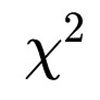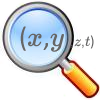#### You may also like### Reaction Timer Timer

How can you time the reaction timer?### Chi-squared Faker

How would you massage the data in this Chi-squared test to both accept and reject the hypothesis?### Chance of That

What's the chance of a pair of lists of numbers having sample correlation exactly equal to zero?

# Very Old Man

##### Age 16 to 18Challenge Level

This problem is can be tackled in a number of different ways - both algebraically and graphically. Because of the different ways of grouping the given data for use graphically, this solution will be solely algebraic.

Using the first two given pieces of data, it can be seen that the proportion of people over the age of 100 who are also over the age of 110 is $\frac{82}{450000}$. Thus, it can be approximated that the probability of a person people over the age of 110, given that they are over the age of 100, is $\frac{82}{450000}$.
$p(Age > 110) = 1 - p(100< Age< 110)$
$= 1-(p + p^2 +p^3 +p^4 +...+p^{10})$

The term shows in brackets is a geometric series, and can be calculated as follows:

Let $X = p +p^2 +p^3 +p^4 +...+p^{10}$
Therefore, $pX = p^2 +p^3 +...+p^{11}$

Subtracting the first of these from the second:

$pX - X = p^{11} -p$
$X(p-1) = p(p^{10} -1)$
$X = \frac{p(p^{10} -1)}{p-1}$

Therefore, using this expression for the geometric series gives:

$p(Age > 110) = 1 - \frac{p(p^{10} -1)}{p-1}$

Since $p(Age > 110) = \frac{82}{450000}$ the equation can be rearranged to give:

$p^{11} -\frac{899918p}{450000} +\frac{449918}{450000} =0$

This polynomial equation is clearly VERY difficult to solve, and so it is best solved numerically. This can be done any number of ways, but is best done using either the Newton-Raphson technique by hand, or by using the 'Solve' function on some calculators, or by using an internet polynomial solver program.

Using these gives a valid root of:
$$p = 0.500...$$

Additional data is given that there are another 31 unverified claims of people over the age of 100. Using this data gives a total 113 people over the age 110, which gives:
$$p = 0.556...$$

A similar calculation can be carried out for people over the age of 115. Using the given data of 2 people over this age gives a polynomial equation:

$p^{16} - \frac{899998p}{450000} + \frac{499998}{450000} = 0$
$$\therefore p = 0.556...$$

Also, using the additional unverified data reveals 4 people over the age of 115, and thus:
$$p = 0.556...$$

From the data so far the values of p are more consistent when including the potentially spurious data, and so it seems justifiable that it should be included. Doing so gives a consistent value of $\mathbf{p =0.556}$.

There are also two final pieces of data given about ages over the last 50 years. This data is somewhat more difficult to use as no data is given for the total number of people that have been aged over 100 years old in the 50 year period. Therefore, the first aim is to estimate the number of total people who have been aged over 100 years:

Firstly, it could be assumed that at any one time there are a constant 450000 people who are aged over 100. Each year, 450000p of these die, and are replaced by the same number. Therefore, over the 50 year period , there is a turnover of $450000 \times 50p$. Thus, the overall number of people over 100 in this time period is give by $450000 + (450000 \times 50p) = 450000(1 +50p)$.

Therefore, for those aged over 115, an equation can be formed:

$\frac{25}{450000(1 +50p)} = 1 - \frac{p(p^{15}-1)}{p-1}$
$50p^{17} + p^{16} - 100p^2 + \frac{864001p}{18000} + \frac{499975}{450000} = 0$
$$p =0.500...$$

For those aged over 120:

$\frac{2}{450000(1+50p)} = 1 - \frac{p(p^{20}-1)}{p-1}$
$50p^{22} + p^{21} -100p^2 + \frac{21600002p}{450000} + \frac{449998}{450000} = 0$
$$p = 0.500...$$

Using this additional data seems to support the exclusion of the unverified data from previously, since both values of p here are the same as that for the original calculation for ages greater than 110. It is difficult to comment on whether it is justifiable to include or exclude the unverified data: its exclusion relies heavily on the calculation for the number of people aged of 100 in the 50 year period as being flawed.

The final part of the question asks what $p(Age > 196)$ is. An equation can be set up as follows:

$p(Age> 196) = 1 - \frac{p(p^{96} -1)}{p-1}$
With a value of $p=0.5$, this yields:

$p(Age > 196) = 2^{-96}$

To calculate the number of people that would need to be in the room before we feel confident that the age of 196 is possible, is given by:

$\frac{1}{2^{-96}} = 7.92 \times 10^{28}$ people in the room.

The total number of people in the world is 6.7 billion, which is $6.7 \times 10^9$, and so we would need $1.2 \times 10^{19}$ times more people than in the whole world to be confident that Li Ching-Yueng's claim is true.